TitleCollege Algebra
Tutorial 30: Introduction to FunctionsLearning Objectives

 After completing this tutorial, you should be able to: Know what a relation, function, domain and range are. Find the domain and range of a relation. Identify if a relation is a function or not. Evaluate functional values.Introduction

 In this tutorial we will be taking a look at working with functions.   We will start off by looking for the domain and range.  Then, we will move on to finding functional values.  Don't be thrown by the different way function notation looks.  When you go to evaluate these functions, we are just plugging in values for x and simplifying, just like you have done a million times before (it is one of those types of problems that I warn students to not make harder than it is).  Just think of finding a functional value as a fancy assignment statement.  I think we are ready to forge ahead.Tutorial

 Relation

 A relation is a set of ordered pairs where the first components of the ordered pairs are the input values and the second components are the output values.

 Function

 A function is a relation that assigns to each input number EXACTLY ONE output number.    Be careful.  Not every relation is a function.  A function has to fit the above definition to a tee.

 Domain

 The domain is the set of all input values to which the rule applies.  These are called your independent variables. These are the values that correspond to the first components of the ordered pairs it is associated with.

 Range

 The range is the set of all output values.  These are called your dependent variables. These are the values that correspond to the second components of the ordered pairs it is associated with.Example 1:  Determine if the given relation is function or not.  Give its domain and range. {(1, 2), (1, -2), (2, 3), (3, 4)}

 Is this a function or not? We need to ask ourselves, does every first element (or input) correspond with EXACTLY ONE second element (or output)?  In this case, the answer is no.  The input value of 1 goes with two output values, 2 and -2.   It only takes one input value to associate with more than one output value to be invalid as a function. So, this relation would not be an example of a function.   Domain We need to find the set of all input values.  In terms of ordered pairs, that correlates with the first component of each one.  So, what do you get for the domain? If you got {1, 2, 3}, you are correct! Note that if any value repeats, we only need to list it one time.   Range We need to find the set of all output values.  In terms of ordered pairs, that correlates with the second component of each one.  So, what do you get for the range? If you got {2, -2, 3, 4}, you are absolutely right!Example 2:  Determine if the given relation is function or not.  Give its domain and range. {(5, 10), (10, 10), (15, 10)}

 Is this a function or not? We need to ask ourselves, does every first element (or input) correspond with EXACTLY ONE second element (or output)?  In this case, the answer is yes.  5 only goes with 10, 10 only goes with 10, and 15 only goes with 10. Note that a relation can still be a function if an output value associates with more than one input value as shown in this example.  But again, it would be a no no the other way around, where an input value corresponds to two or more output values. So, this relation would be an example of a function.   Domain We need to find the set of all input values.  In terms of ordered pairs, that correlates with the first component of each one.  So, what do you get for the domain? If you got {5, 10, 15}, you are correct!     Range We need to find the set of all output values.  In terms of ordered pairs, that correlates with the second component of each one.  SO, what do you get for the range? If you got {10}, you are absolutely right! Note that if any value repeats, we only need to list it one time.Example 3:  Decide whether y is a function of x: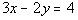.

 To check if y is a function of x, we need to solve for y first and then check to see if there is only one output for every input.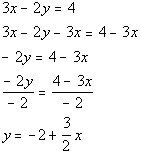*Inverse of add 3x is sub. 3x   *Inverse of mult. by -2y is div. by -2y   *Solved for y

 At this point we ask ourselves, would we get one value for y if you plug in any value for x? If you answered yes, you are right on. For example, if we plugged in a 1 for x, then y would only equal one value, -1/2.  Note that ANY value you would plug in for x would produce only one value for y. Note that since it is solved for y, y is our output value and x is our input value.  Since our answer to that question is yes, that means by definition, y is a function of x.Example 4:  Decide whether y is a function of x: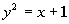To check if y is a function of x, we need to solve for y and then check to see if there is only one output for every input.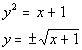*Inverse of squaring is taking the sq. root *Solved for y

 At this point we ask ourselves, would we get one value for y if you plug in any value for x? If you answered no, you are correct.  For example, if our input value x is 3, then our output value y could either be 2 or -2.  Note that I could have picked an infinite number of examples like this one.  You only need to show one example where the input value is associated with more than one output value to disqualify it from being a function. This means that at least one input value is associated with more than one output value, so by definition, y is not a function of x.

 Function Notation f(x) read “f of x”

 f is the function name.  Output values are also called functional values. Note that you can use any letter to represent a function name, f is a very common one used. x is your input variable.  Think of functional notation as a fancy assignment statement.   When you need to evaluate the function for a given value of x, you simply replace x with that given value and simplify.  For example, if we are looking for f(0), we would plug in 0 as the value of x in our function f.Example 5:   Find the functional values f(-2), f(0), and f(1) for the function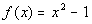.

 Again, think of functional notation as a fancy assignment statement.  For example, when we are looking for f(0), we are going to plug in 0 as the value of x in our function f and so forth.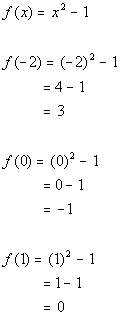*Plug in -2 for x and evaluate       *Plug in 0 for x and evaluate       *Plug in 1 for x and evaluate

 So our answers are f(-2) = 3, f(0) = -1, and f(1) = 0.Example 6:  Find the functional values g(-5), g(0), and g(5) for the function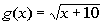.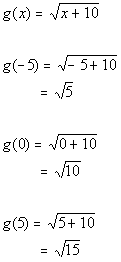*Plug in -5 for x and evaluate     *Plug in 0 for x and evaluate     *Plug in 5 for x and evaluate

 So our answers are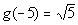,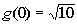, and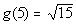.Example 7:  Find and simplify a) f(a),   b) f(a + h) and  c)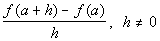using the function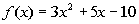.

 f(a): Don't let the fact that we need to plug in an a throw you.  You plug it into the function just like you do a number.  Everywhere you have an x in your function, replace it with an a.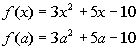*Plug in a for x

 f(a + h) Don't let the fact that we need to plug in the expression a + h throw you.  You plug it into the function just like you do a number.  Everywhere you have an x in your function, replace it with an a + h.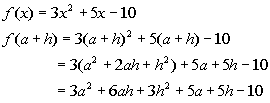*Plug in a + h for x and evaluate: Putting it all together we get: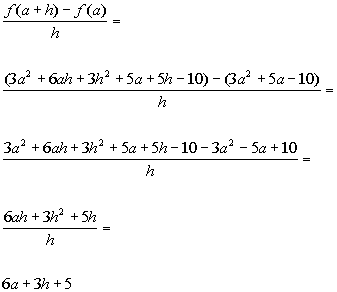*Plug in f( a + h) and f(a) found above           *Combine like terms   *Divide out h from every term

 Constant Function A function of the form  f(x) = C,  where C is a constant.

 If the function is constant, that means that the functional value never changes, it is always equal to that constant.Example 8: Find the functional values h(0) and h(2) of the constant function h(x) = -5.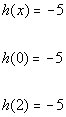Since there is no x to plug into, the functional value is going to be -5 no matter what x is.

 Compound Function

 A compound function is also known as a piecewise function.  The rule for specifying it is given by more than one expression.Example 9: Find the functional values f(1), f(3), and f(4) for the compound function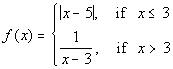To find f(1), we need to go to the piece of the function that x = 1 would be under, which would be the first one where x <  3.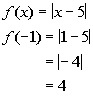*This piece of the function is used when x <  3 *Plug in 1 for x

 To find f(3), we need to go to the piece of the function that x = 3 would be under, which would be the first one where x <  3.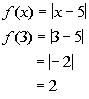*This piece of the function is used when x <  3 *Plug in 3 for x

 To find f(4), we need to go to the piece of the function that x = 4 would be under, which would be the last one where x > 3.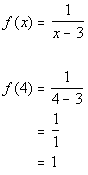*This piece of the function is used when x > 3   *Plug in 4 for x

 Finding the Domain Given an Equation

 As mentioned above, the domain is the set of all input values to which the rule applies.  If you are needing to find the domain given an equation, you want to look for all values of x that would give you a real number answer for your functional value.  A way to approach this is to look to see if there are any restrictions.  If there are none, your domain is all real numbers.  But, sometimes that is not the case.  For example, if your function has a denominator, you have to be careful that the denominator does not equal 0.  You would have to restrict values for x that would cause the denominator to be 0.  Or if you had a square root as part of your function, you would have to be careful to not include values of x that would cause the expression under the square root to be negative.  Let's look at a couple examples to illustrate what I'm talking about here.Example 10:  Give the domain of the function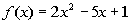.

 Since we can plug in any value for x (input variable) and get a real number answer for our function (output variable), then there are no restrictions.  Therefore the domain is all real numbers.Example 11: Give the domain of the function.

 This time we do have a restriction on our domain.  We can only take the square root of a number greater than or equal to 0. So we must set the radicand (the inside of the radical) greater than or equal to 0.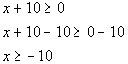*Inv. of add. 10 is sub. 10 *This is our domain

 Only values of x that are greater than or equal to -10 make the original radicand greater than or equal to 0, so x>-10 is our domain for this function.Example 12: Give the domain of the function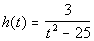.

 Our restriction here is that the denominator of a fraction can never be equal to 0.  So to find our domain, we want to set the denominator equal to 0 and restrict those values.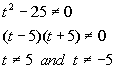*The den. cannot equal to 0 *Factoring to help "solve" *5 and -5 are restricted values

 Our domain would be all real numbers except 5 and -5, because 5 and -5 both make the denominator equal 0, which would not give us a real number answer for our function.Practice Problems

 These are practice problems to help bring you to the next level.  It will allow you to check and see if you have an understanding of these types of problems. Math works just like anything else, if you want to get good at it, then you need to practice it.  Even the best athletes and musicians had help along the way and lots of practice, practice, practice, to get good at their sport or instrument.  In fact there is no such thing as too much practice. To get the most out of these, you should work the problem out on your own and then check your answer by clicking on the link for the answer/discussion for that  problem.  At the link you will find the answer as well as any steps that went into finding that answer.Practice Problems 1a - 1b: Determine if the given relation is function or not.  Give its domain and range.

 1a. {(1, 5), (2, 6), (3, 7), (4, 8)} (answer/discussion to 1a) 1b. {(-1, 2), (-1, 3), (2, 4)} (answer/discussion to 1b)Practice Problems 2a - 2b: Decide whether y is a function of x.

 2a.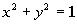(answer/discussion to 2a) 2b.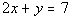(answer/discussion to 2b)Practice Problem 3a: Find f(-5) and f(2) for the given function.

 3a.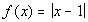(answer/discussion to 3a)Practice Problem 4a: Find and simplify a) f(a),  b) f(a + h) andusing the given function.

 4a.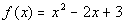(answer/discussion to 4a)Practice Problem 5a: Find the functional values g(-1), g(0), and g(4) for the compound function.

 5a.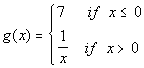(answer/discussion to 5a)Practice Problems 6a - 6c: Give the domain of the function.

 6a.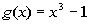(answer/discussion to 6a) 6b.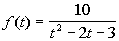(answer/discussion to 6b)

 6c.(answer/discussion to 6c)Need Extra Help on these Topics?

The following are webpages that can assist you in the topics that were covered on this page:

 http://www.wtamu.edu/academic/anns/mps/math/mathlab/int_algebra/int_alg_tut13_func.htm This webpage helps you with the basics of functions. http://www.purplemath.com/modules/fcnnot.htm This website goes over function notation. http://www.purplemath.com/modules/fcns.htm This website goes over what a function is and what domain and range are.

Go to Get Help Outside the Classroom found in Tutorial 1: How to Succeed in a Math Class for some more suggestions.

Last revised on March 30, 2010 by Kim Seward.